# Unbounded sRGB: Levels gamma slider adjustments fail with out of gamut colors

In unbounded sRGB, colors that are out of gamut with respect to the bounded sRGB color space are encoded using at least one negative channel value and might have one or two channel values that are greater than 1.0. For these out of gamut colors, Levels gamma slider adjustments fail completely.

Written April 2014. Updated August 2014.

This article is part of a series of articles on the limitations of unbounded sRGB as a universal color space for image editing.

## Introduction: Levels Channal Value gamma slider adjustments fail in unbounded sRGB

Occasionally a software developer will conclude that because unbounded sRGB (also called "extended sRGB") can be used to encode, display, and store all possible colors, therefore it's also suitable as a "universal working space" for image editing. However, encoding out of gamut colors in the unbounded sRGB color space always requires using one or two negative channel values and might require using one or two channel values that are greater than 1.0. Many editing operations, including Levels gamma slider adjustments, fail completely when done on colors that are encoded using channel values that are less than 0.0 or greater than 1.0.

In case you thinking about 8-bit or 16-bit integer image editing, where of course RGB values are greater than 1.0, gamma calculations are always performed on RGB data that has been normalized. "Normalized" just means you divide all the RGB values by 255 or 65535 as appropriate, perform the gamma calculations, then multiply by 255 or 65535.

I used high bit depth GIMP 2.9 from git to prepare the images shown on this page. High bit depth GIMP from git is somewhat unusual among RGB image editors in that it actually can be used to edit images in the unbounded sRGB color space. The default GIMP 2.9 from git uses linear gamma RGB values for some editing operations and uses "gamma corrected" RGB values for other editing operations. To avoid any accidental "apples to oranges" comparisons, I compiled and used a version of GIMP from git that I modified to ensure that all editing operations were done using linear gamma processing.

Figures 1 and 2 below shows an example of just how badly a simple Levels Value Channel gamma slider adjustment can fail after an image with colors that fall outside the bounded sRGB color gamut is converted to unbounded sRGB: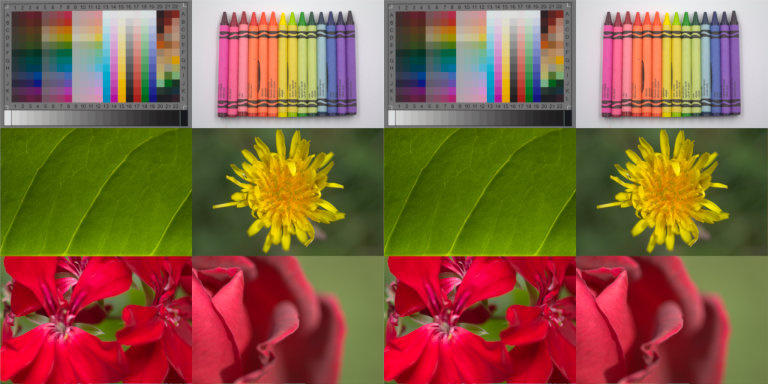Six interpolated camera raw files combined into one composite image (reduced in size, of course). On the left, the image is in a custom camera-sized RGB working space. On the right, the image has been converted to unbounded sRGB. The two images look the same because they are the same. The only difference is the chromaticities used to encode the image colors. Many of the image colors, in particular the green leaf, the yellow dandelion, and the magenta flower colors, are out of gamut with respect to the bounded sRGB color space, and hence are encoded in the unbounded sRGB color space using at least one negative RGB channel value.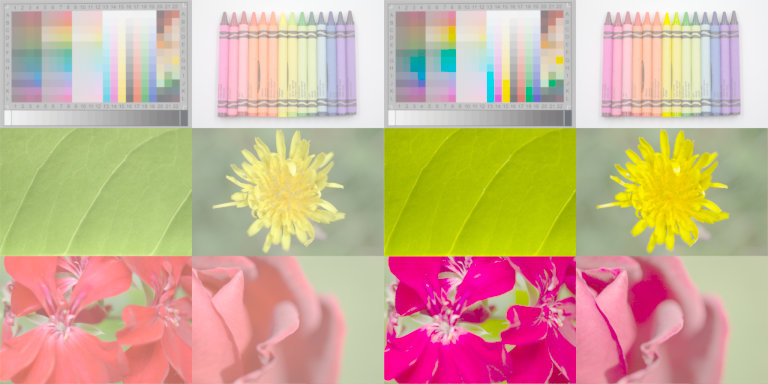The composite image after a Levels Value Channel gamma slider adjustment, moving the gamma slider from 1.00 to 3.00. On the left, the gamma slider adjustment was done in the camera-sized RGB working space. These results are correct. On the right, the gamma slider adjustment was done in the unbounded sRGB color space. These results are very, very wrong. The Levels gamma slider adjustment failed miserably in the unbounded sRGB color space.

As Figures 1 and 2 above illustrate, the fact that colors can be correctly encoded in unbounded sRGB does not mean that all editing operations will work correctly in unbounded sRGB. The mathematics of gamma slider adjustments will explain what went wrong when trying to do a gamma slider correction on out of gamut colors in the unbounded sRGB color space:

## What went wrong in the unbounded sRGB color space?

After converting the image from the custom RGB working space to the unbounded sRGB color space, many RGB values are less than 0.0 and/or greater than 1.0: The green leaf and the dandelion have negative values in the Blue channel. The bright red flowers and the rose have negative values in the Green channel. The rose and the dandelion have Red channel values that are greater than 1.0.

Let's take a closer look at the green leaf and see what happens to the negative channel values after the Levels Channel gamma slider adjustment in the unbounded sRGB color space.

Moving the Levels gamma slider to 3.00 in the Value channel is mathematically equivalent to moving the gamma slider to 3.00 individually in all three color Channels. So moving the gamma slider to 3.00 in just the Blue channel should give the image a pronounced blue color cast. As you can see in Figure 3 below, this is exactly what happens in the custom RGB color space on the left, but not in the unbounded sRGB color space on the right: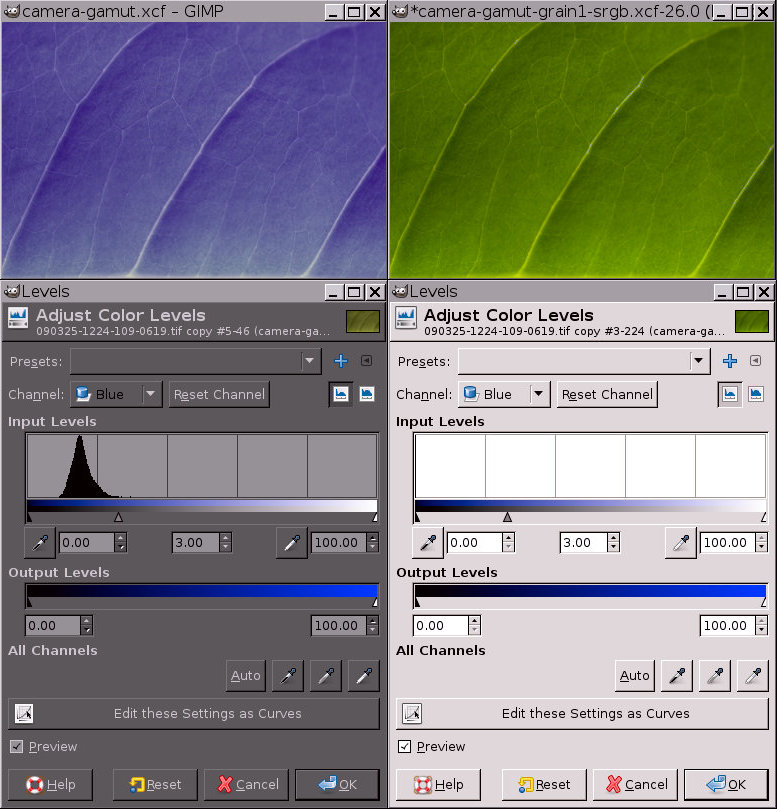Moving the Blue channel gamma slider from 1.00 to 3.00 should make the green leaf turn blue: In the camera-based color space shown above on the left, moving the Blue channel gamma slider from 1.00 to 3.00 did make the green leaf turn blue. In the unbounded sRGB color space shown above on the right, moving the Blue channel gamma slider didn't have any affect at all on the image's color. This is because the GIMP Levels gamma slider adjustment didn't have any effect on the out of gamut RGB channel values that were less than 0.0. Consequently, in the unbounded sRGB color space, moving the Value gamma slider to 3.00 made the image more Green and more Red, but not more Blue. Hence the image acquired a pronounced yellow color cast and is actually more saturated than the original, already saturated green leaf.

You might think that modifying the GIMP Levels gamma slide code to work on negative RGB values might fix the problem. But actually it makes the problem worse:

Figure 4 below compares the result of moving the Levels Value Channel gamma slider from 1.00 to 3.00 in the unbounded sRGB color space, before and after modifying the Levels code to operate on negative Channel values: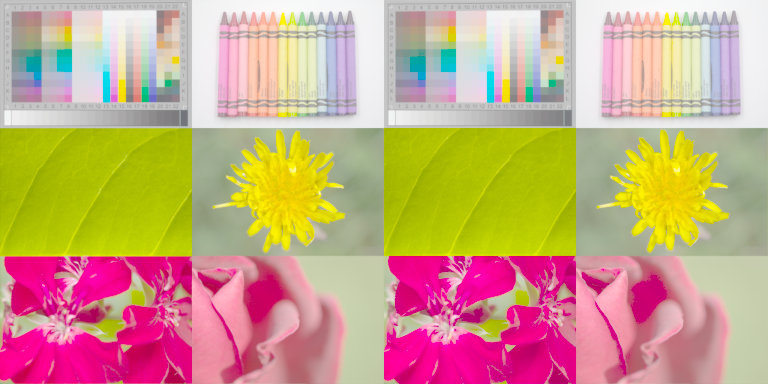Above left: before modifying the Levels code. Above right: after modifying the Levels code. Modifying the Levels code didn't produce much visual change because the resulting colors in either case are out of gamut with respect to what my monitor can display, and also of course with respect to the bounded sRGB color space we use for images displayed on the web. You can't tell just by looking at the images above, but the modified Levels code actually drove the out of gamut negative RGB values even farther out of gamut. However, comparing the rose image on the left (default Levels code) with the rose image on the right (modified Levels code), you can probably see that the modified Levels code significantly increased the splotchiness in the shadow portion of the rose petal on the right.

## The math behind gamma slider adjustments

### The math behind gamma slider adjustments is very simple

The only thing the Levels gamma slider adjustment does is raise the image RGB values to the power of the inverse of the number you select on the gamma slider. That's way more complicated to say than to calculate.

Let's say you have a solid gray linear gamma image with the RGB values (0.5, 0.5, 0.5). Let's say you dial in the gamma slider value "3.00". Here's how the resulting RGB values are calculated:
1. Taking the inverse of 3.00 gives 0.333333.
2. Raising 0.5 to the 0.333333 power (0.5^0.333333) gives 0.793701.

So starting with (0.5,0.5,0.5), the resulting RGB values after you move the gamma slider to 3.00 are (0.793701,0.793701,0.793701).

### The Levels gamma slider adjustment and bounded RGB values

The Levels gamma slider adjustment is designed to work with RGB values that are bounded by 0.0 and 1.0. Within this bounded range, gamma slider adjustments have:

• No effect at all on RGB values that are equal to 0.0 or 1.0
• Maximum affect on RGB values that are equal to some number midway between 0.0 and 1.0, and progressively less effect as the RGB values get farther from that number:
1. For gamma=0.5, the "midway" number is 0.5.
2. For gamma=2.0, the "midway" number is 0.25.
3. For gamma=3.0, the "midway" number is approximately 0.19.
4. For gamma=4.0, the "midway" number is 0.16.

### The Levels gamma slider adjustment and unbounded RGB values

If an image has colors that exceed the very small bounded sRGB color gamut, converting the image to unbounded sRGB necessarily produces RGB values that are less than 0.0 and/or greater than 1.0. So the results of gamma slider adjustments are no longer confined to the bounded range between 0.0 and 1.0. Rather for input RGB values >1.0, output RGB values spiral upwards past 1.0. And for input RGB values <0.0, output RGB values spiral downward below 0.0. This is why the out of gamut colors produce even farther out of gamut colors after a gamma slider adjustment is done, if the clipping code is removed. The table below gives some sample calculations:

The default GIMP Levels code doesn't (and shouldn't!) modify any RGB values that are less than 0.0:

```if (inv_gamma != 1.0 && value > 0)
value =  pow (value, inv_gamma);
```

You can't actually calculate the fractional power of a value that's less than 0.0. So when raising a negative number to a power between 0.0 and 1.0, the usual procedure is to multiply the negative values by -1.0 to make them positive, calculate the power, then multiply by -1.0 again to make the resulting values negative again:

```if (inv_gamma != 1.0 && value < 0)
{
value = -1.0 * value;
value =  pow (value, inv_gamma);
value = -1.0 * value;
}
```

The Table below shows how the results of gamma slider adjustments spiral upwards and downwards when applied to out of gamut RGB channel values, with and without a code modification to allow calculating the result of raising a negative channel value to a fractional power:

Gamma slider adjustments produce increasingly out of gamut colors for unbounded RGB values
input RGB value Gamma=3.00
(default code)
Gamma=0.33
(default code)
Gamma=3.00
(modified code)
Gamma=0.33
(modified code)
8.000000 2.000000 545.301037 2.000000 545.301037
4.000000 1.587400 66.745842 1.587400 66.745842
2.000000 1.259921 8.169813 1.259921 8.169813
1.500000 1.144714 3.416724 1.144714 3.416724
1.000000 1.000000 1.000000 1.000000 1.000000
0.750000 0.908560 0.418213 0.908560 0.418213
0.500000 0.793701 0.122402 0.793701 0.122402
0.250000 0.629961 0.014982 0.629961 0.014982
0.000000 0.000000 0.000000 0.000000 0.000000
-0.500000 0.500000 -0.500000 -0.793701 -0.122402
-1.000000 -1.000000 -1.000000 -1.000000 -1.000000
-1.500000 -1.500000 -1.500000 -1.144714 -3.416724
-2.000000 -2.000000 -2.000000 -1.259921 -8.169813
-4.000000 -4.000000 -4.000000 -1.587400 -66.745842
-8.000000 -8.000000 -8.000000 -2.000000 -545.301037

## Conclusion: the mathematics of gamma slider adjustments only works with bounded RGB data

Gamma slider adjustments are designed to work with RGB channel values that are bounded by 0.0 and 1.0. Therefore, in the unbounded sRGB color space, Levels Value Channel gamma slider adjustments fail for images with channel values that are less than 0.0 or greater than 1.0:

In the original custom RGB working space where all channel values are between 0.0 and 1.0 inclusively, gamma slider adjustments work as expected:

• Before and after the gamma slider adjustment, all the RGB values fall within the range of 0.0 to 1.0.
• After the gamma slider adjustment, all the RGB values have been raised to the power of 0.333333 (the inverse of 3.00), and consequently the resulting image is both lighter in tonality and also everywhere less saturated than the original image.

In the unbounded sRGB color space, the mathematics of gamma slider adjustments is completely messed up:

• Before and after the gamma slider adjustment, the out of gamut colors have RGB channel values are either negative or greater than 1.0.
• After the gamma slider adjustment, the positive RGB values are all made brighter. Any RGB channel values that are greater than 1.0 are made far too bright. But the negative RGB values are left unchanged if using the unmodified Levels code, and made even more negative if using the modified Levels code. Consequently, anywhere the image RGB values are negative, after a gamma slider adjustment the resulting image is considerably more saturated than it was before the gamma slider adjustment.

As a very important aside, Curves are an extension of Levels. As Levels gamma slider adjustments fail in unbounded sRGB, Curves will fail for the same reasons.

## Appendix: Compressing the data before performing the gamma slider adjustment doesn't work

Converting an image from a wider gamut RGB working space to unbounded sRGB can easily result in RGB values that are outside the bounded 0.0 to 1.0 range. It has been suggested that the solution to editing problems caused by channel data that is outside the bounded 0.0 to 1.0 range is to:

1. Compress the data to fit within the bounded range 0.0 to 1.0.
2. Perform the operation.
3. Uncompress the data back to the original range.

However, as Figures 5 through 7 below show, converting to unbounded sRGB, then compressing the data to fit within the bounded range 0.0 to 1.0 before making a gamma slider adjustment, then uncompressing the result, does not produce the same result as making the gamma slider adjustment in the original color space: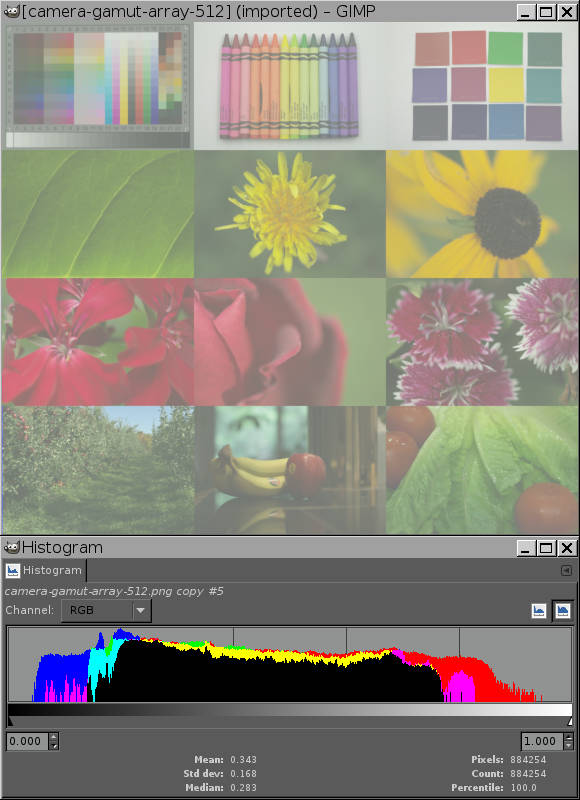The same image shown in Figure 1 above on the left, except now the Levels lower left and right sliders have been used to compress the data to fit inside the sRGB bounded color gamut.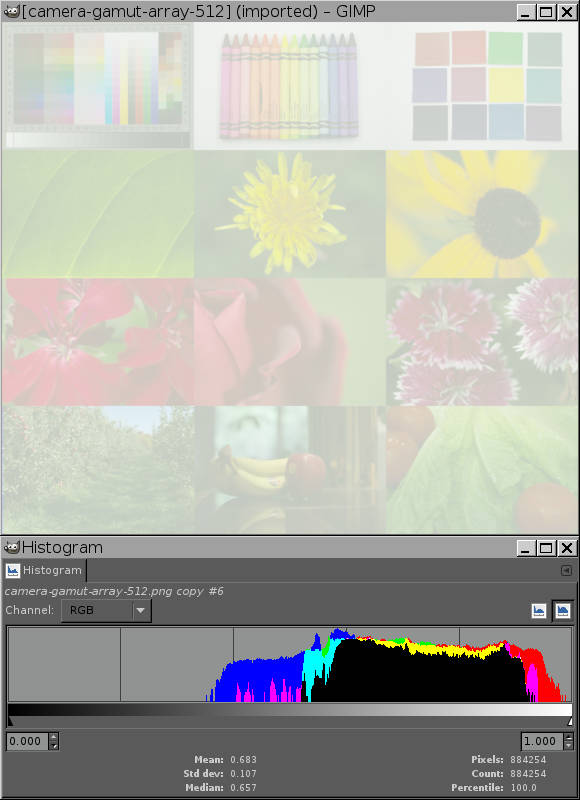Having been compressed to fit within the sRGB bounded color gamut, now the image is given a Value channel gamma slider adjustment of gamma=3.0, just as in Figure 2 above. The suggestion is that compressing the data before doing the gamma slider adjustment might mean the resulting image is not damaged by using the gamma slider adjustment in the unbounded sRGB color space. Alas Figure 7 below shows that this is not the case.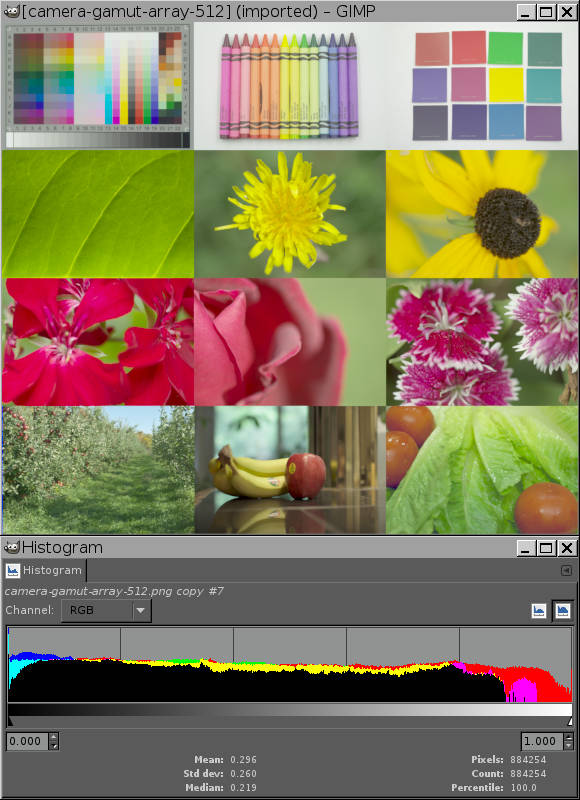Now the image has been uncompressed back to the original range. The image has been nicely brightened in the highlights but this isn't what the goal actually was. The goal was to convert from the source color space to unbounded sRGB and then use the Levels gamma slider to produce an image that looks like the image in Figure 2 above on the left.